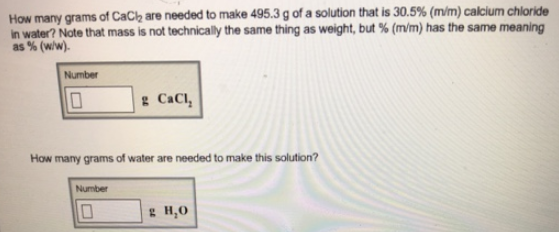# Problem: How many grams of CaCl2 are needed to make 495.3 g of a solution that is 30.5% (m/m) calcium chloride in water? Note that mass in not technically the same thing as weight, but % (m/m) has the same meaning as % (w|w). How many grams of water are needed to make this solution?

###### FREE Expert Solution
81% (246 ratings)###### Problem Details

How many grams of CaCl2 are needed to make 495.3 g of a solution that is 30.5% (m/m) calcium chloride in water? Note that mass in not technically the same thing as weight, but % (m/m) has the same meaning as % (w|w).

How many grams of water are needed to make this solution?